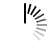# Shuffle Array Contents

This algorithm will take an array and shuffle the contents of the array. It will generate a random permutation of the array elements.

To solve this problem, we will swap elements starting from the last index to randomly generated an index in the array.

#### Input and Output

```Input:
An array of integers: {1, 2, 3, 4, 5, 6, 7, 8}
Output:
Shuffle of array contents: 3 4 7 2 6 1 5 8 (Output may differ for next run)```

#### Algorithm

`randomArr(array, n)`

Input: The array, number of elements.

Output: Shuffle the contents of the array.

```Begin
for i := n – 1 down to 1, do
j := random number from 0 to i
swap arr[i] and arr[j]
done
End```

#### Source Code (C++)

```#include <iostream>
#include<cstdlib>
#include <ctime>
using namespace std;

void display(int array[], int n) {
for (int i = 0; i < n; i++)
cout << array[i] << " ";
}

void randomArr ( int arr[], int n ) {           //generate random array element
srand (time(NULL));                 //use time to get different seed value to start
for (int i = n-1; i > 0; i--) {
int j = rand() % (i+1);           //randomly choose an index from 0 to i
swap(arr[i], arr[j]);            //swap current element with element placed in jth location
}
}

int main() {
int arr[] = {1, 2, 3, 4, 5, 6, 7, 8};
int n = 8;
randomArr(arr, n);
display(arr, n);
}```

#### Output

`4 7 8 2 6 3 5 1`